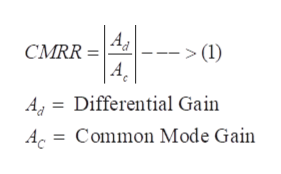# An opamp is having a differential gain Ad =4500 and a common mode gain Ac = 25. What will be value of Common Mode Rejection Ratio (CMRR)? Express your answer in decibels (dB).

Question
1 views

An opamp is having a differential gain Ad =4500 and a common mode gain Ac = 25. What will be value of Common Mode Rejection Ratio (CMRR)? Express your answer in decibels (dB).

check_circle

star
star
star
star
star
1 Rating
Step 1

For an Op-amp, common mode rejection ratio (CMRR) is defined as the ratio of the magnitude of the differential gain to the magnitude of the common mode gain. It can be expressed in the following way:help_outlineImage TranscriptioncloseCMRR > (1) A |L A = Differential Gain Ac = Common Mode Gain fullscreen
Step 2

So, in this case, value of CMRR will be

Step 3

When expressed in decibels (dB), ...

### Want to see the full answer?

See Solution

#### Want to see this answer and more?

Solutions are written by subject experts who are available 24/7. Questions are typically answered within 1 hour.*

See Solution
*Response times may vary by subject and question.
Tagged in

### Electrical Engineering